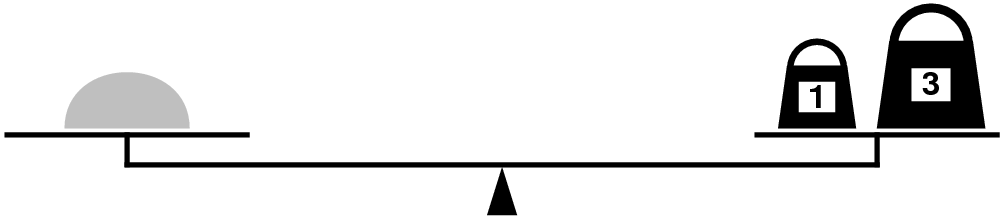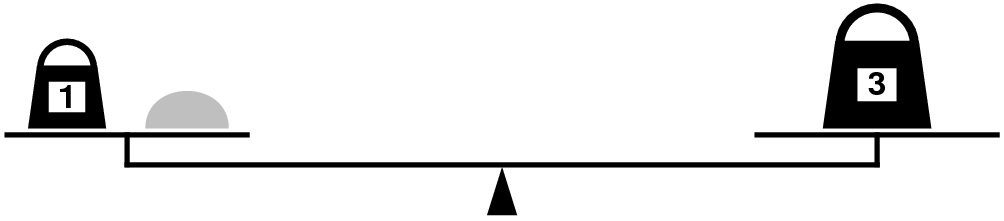#CodeStepByStep## isMeasurable

Language/Type: C++ recursion backtracking

Write a recursive function named `isMeasurable` that determines whether it is possible to weigh out exactly a given amount on two scales, given a target amount and a collection of weights to use. Your function accepts two parameters: a reference to a vector of integers representing weights that you can use, and an integer target weight amount.

For example, suppose that you have only two weights: a 1-ounce weight and a 3-ounce weight. With these you can easily measure out 4 ounces by placing both weights on one side of the scale, as shown:You can also measure out 2 ounces by shifting the 1-ounce weight to the other side, as follows:Suppose that the variable `weights` has been initialized as follows:

```vector<int> weights {1, 3};
```

Given these values, the calls of `isMeasurable(weights, 4)` and `isMeasurable(weights, 2)` would both return `true`. On the other hand, the call of `isMeasurable(weights, 5)` would return `false` because it is impossible to use the 1- and 3-ounce weights to add up to 5 ounces. You don't need to use every weight in the vector to reach the given target; in the example above, calls of `isMeasurable(weights, 3)` and `isMeasurable(weights, 1)` would return `true` because you can make those amounts by using only a single weight in each case.

If the target weight passed is 0, your function should always return `true`. You may assume that the value of the target weight passed will not be initially negative, and that the vector passed does not contain any negative weight values in it.

Constraints: You may use a loop in your solution if you like, but the overall algorithm must use recursion and backtracking. You may define helper functions if you like.

Function: Write a C++ function as described, not a complete program.